Graphical Interpretation of Goal Programming[Page 394 ( continued )]

In Chapter 2 we analyzed the solution of linear programming models by using graphical analysis. Because goal programming models are linear, they can also be analyzed graphically. The original goal programming model for Beaver Creek Pottery Company, formulated at the beginning of this chapter, will be used as an example: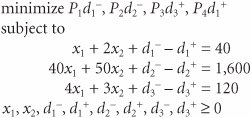To graph this model, the deviational variables in each goal constraint are set equal to zero, and we graph each subsequent equation on a set of coordinates, just as in Chapter 2. Figure 9.1 is a graph of the three goal constraints for this model.

[Page 395]
Figure 9.1. Goal constraints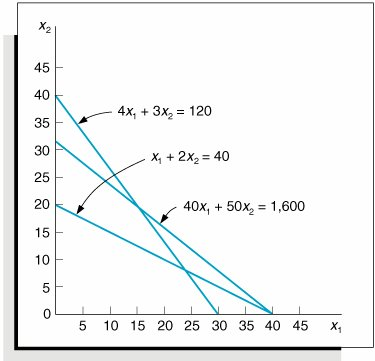Notice that in Figure 9.1 there is no feasible solution space indicated, as in a regular linear programming model. This is because all three goal constraints are equations ; thus, all solution points are on the constraint lines.

The solution logic in a goal programming model is to attempt to achieve the goals in the objective function, in order of their priorities. As a goal is achieved, the next highest-ranked goal is then considered . However, a higher-ranked goal that has been achieved is never given up in order to achieve a lower-ranked goal.

Graphical solution illustrates the goal programming solution logicseeking to achieve goals by minimizing deviation in order of their priority .

In this example we first consider the first-priority goal, minimizing d 1 . The relationship of d 1 and d 1 + to the goal constraint is shown in Figure 9.2. The area below the goal constraint line x 1 + 2 x 2 = 40 represents possible values for d 1 , and the area above the line represents values for d 1 + . In order to achieve the goal of minimizing d 1 , the area below the constraint line corresponding to d 1 is eliminated, leaving the shaded area as a possible solution area.

Figure 9.2. The first-priority goal: minimize d 1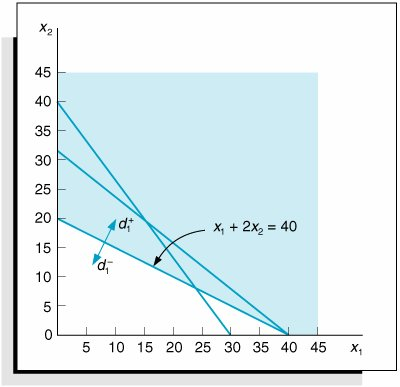[Page 396]

Next, we consider the second-priority goal, minimizing d 2 . In Figure 9.3, the area below the constraint line 40 x 1 + 50 x 2 = 1,600 represents the values for d 2 , and the area above the line represents the values for d 2 + . To minimize d 2 , the area below the constraint line corresponding to d 2 is eliminated. Notice that by eliminating the area for d 2 , we do not affect the first-priority goal of minimizing d 1 .

Figure 9.3. The second-priority goal: minimize d 2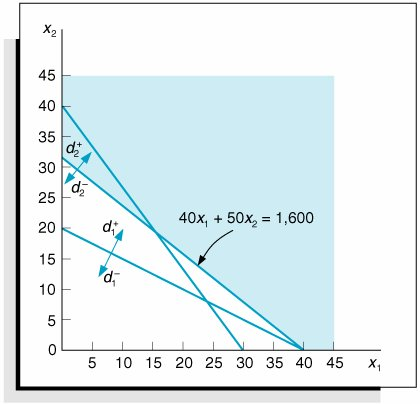One goal is never achieved at the expense of another higher-priority goal .

Next, the third-priority goal, minimizing d 3 + , is considered. Figure 9.4 shows the areas corresponding to d 3 and d 3 + . To minimize d 3 + , the area above the constraint line 4 x 1 + 3 x 2 = 120 is eliminated. After considering the first three goals, we are left with the area between the line segments AC and BC , which contains possible solution points that satisfy the first three goals.

Figure 9.4. The third-priority goal: minimize d 3 +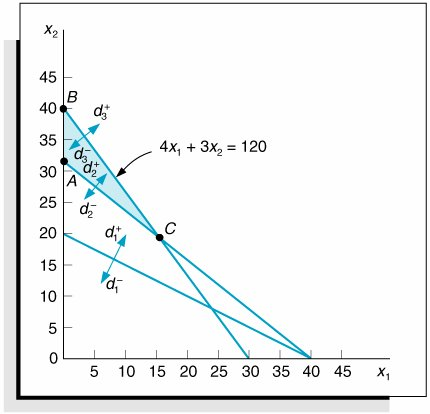Finally, we must consider the fourth-priority goal, minimizing d 1 + . To achieve this final goal, the area above the constraint line x 1 + 2 x 2 = 40 must be eliminated. However, if we eliminate this area, then both d 2 and d 3 must take on values. In other words, we cannot minimize d 1 + totally without violating the first- and second-priority goals. Therefore, we want to find a solution point that satisfies the first three goals but achieves as much of the fourth-priority goal as possible.

[Page 397]

Point C in Figure 9.5 is a solution that satisfies these conditions. Notice that if we move down the goal constraint line 4 x 1 + 3 x 2 = 120 toward point D , d 1 + is further minimized; however, d 2 takes on a value as we move past point C . Thus, the minimization of d 1 + would be accomplished only at the expense of a higher-ranked goal.

Figure 9.5. The fourth-priority goal (minimize d 1 + ) and the solution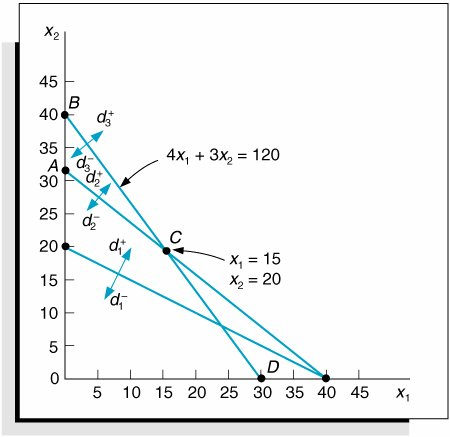The solution at point C is determined by simultaneously solving the two equations that intersect at this point. Doing so results in the following solution: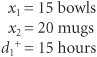Because the deviational variables d 1 , d 2 , and d 3 + all equal zero, they have been minimized, and the first three goals have been achieved. Because d 1 + = 15 hours of overtime, the fourth-priority goal has not been achieved. The solution to a goal programming model such as this one is referred to as the most satisfactory solution rather than the optimal solution because it satisfies the specified goals as well as possible.

Goal programming solutions do not always achieve all goals, and they are not optional; they achieve the best or most satisfactory solution possible .Introduction to Management Science (10th Edition)
ISBN: 0136064361
EAN: 2147483647
Year: 2006
Pages: 358

Similar book on Amazon